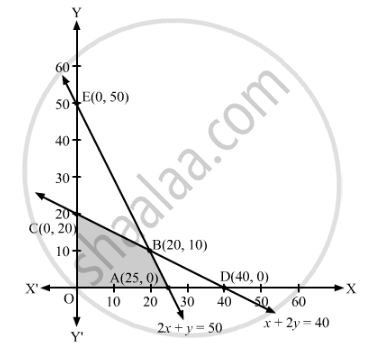# One Kind of Cake Requires 200 G of Flour and 25 G of Fat, and Another Kind of Cake Requires 100 G of Flour and 50 G of Fat. - Mathematics

Sum

One kind of cake requires 200 g of flour and 25 g of fat, and another kind of cake requires 100 g of flour and 50 g of fat. Find the maximum number of cakes which can be made from 5 kg of flour and 1 kg of fat assuming that there is no storage of the other ingredients used in making the cakes.

#### Solution

Let the number of cakes of one kind and another kind be x and y, respectively.

Therefore, the total number of cakes produced are (x + y).

One kind of cake requires 200 g of flour and another kind of cake requires 100 g of flour. So, x cakes of one kind and cakes of another kind requires (200x + 100y) g of flour.  But, cakes should contain atmost 5 kg of flour.

∴ 200x + 100≤ 5000

⇒ 2x + ≤ 50

One kind of cake requires 25 g of fat and another kind of cake requires 50 g of fat. So, x cakes of one kind and cakes of another kind requires (25x + 50y) g of fat.  But, cakes should contain atmost 1 kg of fat.

∴ 25x + 50≤ 1000

⇒ x + 2≤ 40

Thus, the given linear programming problem is

Minimise Z = x + y

subject to the constraints

2x + ≤ 50

x + 2≤ 40

x, y ≥ 0

The feasible region determined by the given constraints can be diagrammatically represented as,The coordinates of the corner points of the feasible region are O(0, 0), A(25, 0), B(20, 10) and C(0, 20).

The value of the objective function at these points are given in the following table.

 Corner Point Z = x + y (0, 0) 0 + 0 = 0 (25, 0) 25 + 0 = 25 (20, 10) 20 + 10 = 30       → Maximum (0, 20) 0 + 20 = 20

Thus, the maximum value of is 30 at x = 20, y = 10.

Hence, the maximum number of cakes which can be made are 30.

Concept: Graphical Method of Solving Linear Programming Problems
Is there an error in this question or solution?

#### APPEARS IN

RD Sharma Class 12 Maths
Chapter 30 Linear programming
Exercise 30.3 | Q 11 | Page 40